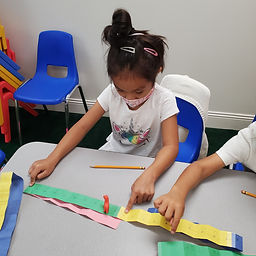## Ms. Raejean

### Target 1​

###### Lesson Type:

New

Number Operation

:

Integer Composition

Use place-value understandings to round whole numbers to the nearest 10.

###### 1:

Determine whether to round up or down to get to the nearest ten.

2nd

###### Vocabulary:

Rounding, Multiples of Ten, "Closest to"

Activities:

Rounding numbers was introduced. We identified the muliples of 10 (10, 20, 30, 40, 50, etc.). Then we used a large number line to round a few numbers to the nearest 10. Next, students constructed their own number line (0-40) to practice rounding numbers. Students found the number on their number line, identified the two nearest 10s, and determined which 10 the number was closest to. Finally, students practiced rounding larger numbers (ex. 56 & 93) on a given number line. (ex. Find 56 on a number line 50 to 60. Then choose the ten it's closest to.)### Home Exploration

###### Guiding Questions:## Absent Students:

### Target 2

:

###### 1:

Use a classification key.

###### 2:

In a given group of objects, determine which objects does not fit with the others.

###### 3:

Name the property that objects have in common.

2nd

###### Vocabulary:

Classify, Common

Activities:

Students looked at a given set of objects and determined which item didn't belong. Then students identified what the remaining shapes had in common.

Next, we used a classification key to determine the name of each character.### Home Exploration

###### Guiding Questions:### Target 3

:

###### Vocabulary:

Activities:### Home Exploration